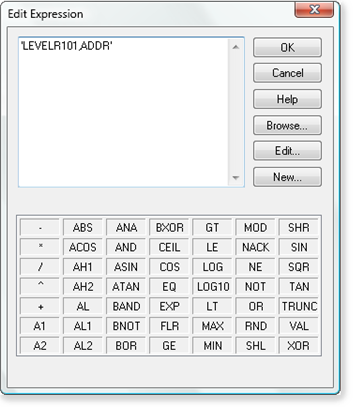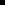#### 1.2. Use Expression Editor OperationsEdit expression operations are available in the Edit Expression dialog box.

 Arithmetic operations. Bitwise operations. Alarm functions. Conversion operation. Logical operations. Relational operations. Scientific operations.

Arithmetic Operations

 Subtraction Multiplication Division The result of dividing two integers in an expression will be an integer. If you want a floating point result, multiply the numerator by 1.0 before dividing. Example POINTA Set to 6. POINTB Set to 4. Result of the expressionPOINTA/POINTB is 1(POINTA*1.0)/POINTB is 1.5. Addition Value of X raised to the power of Y. Takes the square root of an expression. Format SQR expr Takes the absolute value of an expression. Format ABS (expr) Example ABS (-2.6) return 2.6 Returns the minimum value of two expressions. Format (expr1) MIN (expr2) Example 3 MIN 4 returns 3. Returns the maximum value of two expressions. Format (expr1) MAX (expr2) Example 3 MAX 4 returns 4. Returns the modulus value of an expression. Format (expr1) MOD (expr2) Example 9 MOD 8 returns a value of 1. Rounds a floating-point expression to the nearest integer. Format RND (expr) ExampleRND (2.6) returns a value of 3.RND (-2.6) returns a value of -3. Truncates a floating-point expression to its integer value. Format TRUNC (expr) ExampleTRUNC (2.6) returns a value of 2.TRUNC (-2.6) returns a value of -2. Rounds a floating-point expression to the nearest integer that is smaller than the expression. Format FLR (expr) ExampleFLR (2.6) returns a value of 2.FLR (-2.6) returns a value of -3. Rounds a floating-point expression to the nearest integer that is larger than the expression. Format CEIL (expr) ExampleCEIL (2.3) returns a value of 3.CEIL (-2.3) returns a value of -2.

Bitwise Operations

You can use Boolean, integer or floating point number for Bitwise operations. If a number/expression is in floating point, it is rounded off to the nearest integer for these operations.

 Performs bitwise AND of two expressions. Format AND Performs bitwise OR of two expressions Format OR Performs bitwise NOT of an expression Format NOT Performs bitwise XOR of two expressions. Format XOR Performs binary left shift on an expression. Format (expr1) SHL (expr2) Example 2 SHL 1 returns a value of 4. Note: When SHL goes out of range the:Point becomes unavailable.Point Control Pane is starred.Core status log notes that it is unavailable. Performs a binary right shift on an expression. Format (expr1) SHR (expr2) Example 2 SHR 1 returns a value of 1 Note: When SHR goes out of range the:Point becomes unavailable.Point Control Pane is starred.Core status log notes that it is unavailable.

Alarm functions

Alarm functions supported by the Expression Editor are:

 Returns TRUE if the point is in any Alarm or Warning state. Format AL() A1 or WARNING Returns TRUE if the point is in a Warning High or Warning Low state. Format A1() A2 or ALARM Returns TRUE if the point is in an Alarm High or Alarm Low state. Format A2() AH1 or WARNING_HIGH Returns TRUE if the point is in a Warning High state. Format AH1() AH2 or ALARM_HIGH Returns TRUE if the point is in an Alarm High state. Format AH2() AL1 or WARNING_LOW Returns TRUE if the point is in a Warning Low state. Format AL1() AL2 or ALARM_LOW Returns TRUE if the point is in an Alarm Low state. Format AL2() ANA or ALARM_NOT_ACKED Returns TRUE if the point is in alarm and the alarm has not been acknowledged. Note: The ANA behavior was changed in CIMPLICITY V.6.1. Prior to V.6.1 it behaved as NACK. The global parameter PTEXP_ANA_EQ_NACK_AND_AL is available to revert ANA behavior to pre-version 6.. Format ANA() Important: ANA is not supported for Enterprise points. Returns TRUE if the alarm has not been acknowledged, whether or not the point is in an alarm state. Format NACK ()

Conversion operation

 Convert a variable that consists of numbers in text string format to a numeric format that can be included in calculations.

Logical operators

You can use Boolean, integer or floating point numbers for logical operations. If an expression has a non-zero value, it is TRUE; if the value is zero, it is FALSE.

 Performs Logical AND of two expressions. Format AND Performs Logical if A is TRUE, then B, else C. Format If (expr1) THEN (expr2) ELSE (expr3) Or (expr1) ? (expr2) : (expr3) Note: The IF…ELSE statement is not available from the operation keys. However, you can enter it manually. Performs Logical OR of two expressions. Format OR Performs Logical NOT of an expression. Format NOT Performs Logical XOR of two expressions.

Relational operations

 Less Than or Below Format (expr1) LT (expr2 Greater Than or Above. Format (expr1) GT (expr2) Equal To Format (expr1) EQ (expr2) Less Than or Equal To Format (expr1) LE (expr2) Greater Than or Equal To Format (expr1) GE (expr2) Not Equal To Format (expr1) NE (expr2)

Scientific operations

 Returns sine (angle in radians) of an expression. Format SIN (expr) Returns cosine (angle in radians) value of an expression. Format COS (expr) Returns tangent (angle in radians) of an expression. Format TAN (expr) Returns Arc sine (angle in radians) value of an expression. Format ASIN (expr) Returns Arc cosine (angle in radians) value of an expression. Format ACOS (expr) Returns arc tangent (angle in radians) value of an expression. Format ATAN (expr) Raise a value to a power. Format ^ () Returns exponential (ex) value of an expression where x is the expression. Format EXP (expr) Returns natural logarithm (base e) of an expression. Format LOG (expr) Returns base 10 logarithm of an expression. Format LOG10 (expr)
 1. Expression Editor in CimEdit.